# Selina Concise Mathematics Class 6 ICSE Solutions Chapter 6 Negative Numbers and Integers

## Selina Concise Mathematics Class 6 ICSE Solutions Chapter 6 Negative Numbers and Integers

Selina Publishers Concise Mathematics Class 6 ICSE Solutions Chapter 6 Negative Numbers and Integers

### Negative Numbers and Integers Exercise 6 – Selina Concise Mathematics Class 6 ICSE Solutions

Question 1.
Fill in the blanks :
(i) Negative of -20 is ……….
(ii) Negative of 0 is …………
(iii) Negative of 8 is ………..
(iv) If 10 represents gain of ₹ 10, then -10 represents …………..
(v) If going south is negative then going north is …………
(vi) Because 5 < 7, therefore -5 ……….. -7. (vii) If 3 > -2, then 3 is on the ………….. of -2.
(viii) If -8 < -6, then -8 is on the …………. of-6.
Solution: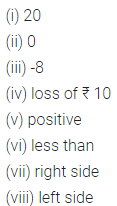Question 2.
Use a number line to write the following integers in ascending (increasing) order :
(i) -5, 8, 0, -9, 4, -14 and 12
(ii) -6, 7, 0, -9, 5 and 9
Solution:Question 3.
Use a number line to write the following integers in descending (decreasing) order :
(i) -10, 0, 3, -4, 12, 11, -1 and 5
(ii) -4, 3, -8, -12, -7 and 6.
Solution: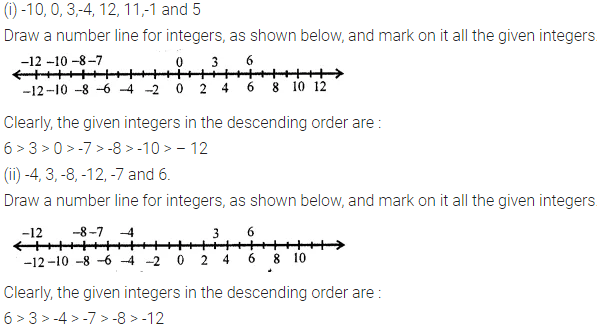Question 4.
(i) 13 and 15
(ii) -13 and 15
(iii) 13 and -15
(iv) -13 and -15
Solution: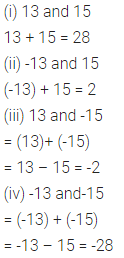Question 5.
(i) 259 from 214
(ii) -528 and -243
(iii) -623 and 326
(iv) 258 and -473
(v) -622 and -254
(vi) 257 and -254
Solution: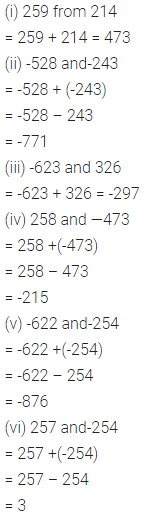Question 6.
Subtract :
(i) 5 from 8
(ii) -5 from 8
(iii) 4 from -7
(iv) -8 from -2
(v) -3 from 12
(vi) -6 from -3
Solution: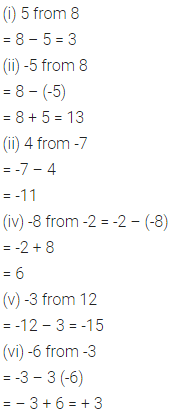Question 7.
Subtract:
(i) -123 from 453
(ii) -78 from -12
(iii) 329 and -124
(iv) -222 from 0
Solution:Question 8.
Using a number line, find the integer which is :
(i) 3 more than -1
(ii) 5 less than 2
(iii) 5 more than -9
(iv) 4 less than -4
(v) 7 more than 0
(vi) 7 less than -8
Solution: